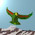## Thursday, December 15, 2011

### Through The Looking Glass III

In 1995 I was in Adelaide attending mathematics workshops on topological field theories. The excellent speakers included Lisa Jeffrey and Frances Kirwan. One day, a few of us went to the pub. Vaughan Jones was talking about knots and subfactors, and in particular the values in the subfactor index series that are less than $4$, given by

$4 \cos^{2} \frac{\pi}{n}$

for $n \geq 3$ an ordinal. I was sitting next to Professor Jones at the pub, and very keen to understand knots, so I asked him where the coefficient of $4$ came from. A local mathematician immediately scoffed at my stupid question, but Professor Jones frowned and thought for a minute, and finally he said: I think the $4$ is the $4$ of the four color theorem. So let us look once again at the successful Higgs mass prediction by Dharwadker and Khachatryan,

$M = \frac{1}{2}(m_{W^{+}} + m_{W^{-}} + m_{Z})$

Observe that $2 M$ is $250$ GeV, the electroweak scale. Fourier twisting the $(W^{+}, W^{-}, Z)$ triplet gives a $(Z,Z,Z)$ color triplet, and dualising this triplet we get the right handed $(e^{+}, e^{-}, \nu)$. The left handed triplet comes from $(W^{+}, W^{-}, \gamma)$, which we can send to the $(e^{+}, e^{-}, \gamma)$ of creation and annihilation with a mixture of Fourier duals. Three massive (weak interaction) bosons and three (electromagnetic) particles, two sides of a coin.

1.And Alejandro now notes that the top quark mass $m_t$ satisfies

$(m_t)^2 = M^2 + m_{W}^2 + m_{Z}^2$

2.Kea,

I thought about it some before I checked your link on such methods predicting "Higgs mass". This was a very interesting and wonderful paper before seeing it I thought I would comment that this direction your post presented seemed a fresh new promising path.

Of course we need to see these things in all the existing models- beginning with the various quantum ideas. There is more to the theory than Riemann's plane alone. We know we can divide the torus surface into seven and its volume into 13 pieces (so models just based on toric lattices are not complete).

If we use some of these ideas, string like actually in the formalism, as when one actually tries to draw out and color maps of a few locally (not proven thereby for very large infinity of regions maps), the novice will come up to five colors sometimes seeming to be needed. The partition number for 4 objects we recall is five.

I am glad you suggest a sort of mirror equivalence here (a model to resolve it somehow directly as in your first paper so to make a clear and obvious assertion with authority)- why after all did Carroll mention Alice in the Looking glass that the fire in her fireplace there was of a different energy? In a sense he foresaw quantum effects.

These ideas, gauge and so on, need to be better refined in the proper dimensions (I would not just base things on the wave mechanics cited by the article, three and one and the change of sign when 4 and 4 work just as well to describe axes and mirrors.

Professor Jones may have had a fine intuition he was not aware of consciously of which the student, if sensitive and creative, can think about and pick up on - thank you for that delightful story.

The PeSla who refrained from stating again on the Higgs news blogs that you are obviously on a better if not the right track.

(ps this is long for your blog so I will include it on mine. If such a paper as you linked to does not have the theoreticians questioning their standard conclusions what can?)

3.Hi, The Pe Sla. Of course I feel like a complete idiot for raving on about fairies all these years, but then everyone is stupid. This four colour thing smells right. And I bet this is the only decent correct prediction out there.

4.Well, the mass of the top quark, or the vacuum 246.21 GeV divided by sqrt(2), it could be any of them. Or it could be neither; it is amusing that simple addition of W and Z does the trick.

Incidentaly, the sum of squares formula is because of Lubos :-D. He mocked of the 4-colours formula and he dismissively told something as "in any case, it should be with the squares of masses", and then I noticed, damn, it is true, it ALSO works something with the squares of masses.

Note: Only a member of this blog may post a comment.